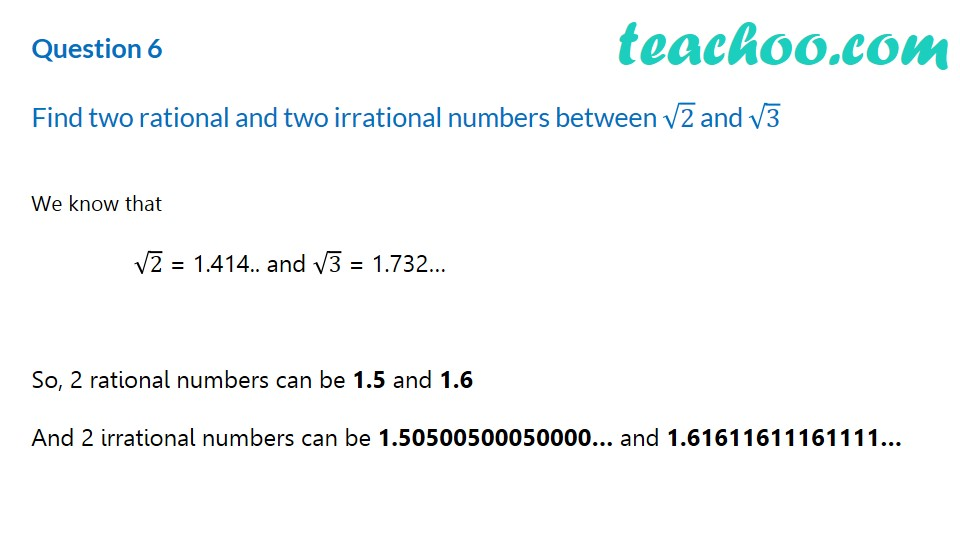Finding irrational numbers b/w two numbers

Chapter 1 Class 9 Number Systems
Concept wiseLearn in your speed, with individual attention - Teachoo Maths 1-on-1 Class

### Transcript

Question 6 Find two rational and two irrational numbers between √2 and √3 We know that √2 = 1.414.. and √3 = 1.732… So, 2 rational numbers can be 1.5 and 1.6 And 2 irrational numbers can be 1.50500500050000… and 1.61611611161111…